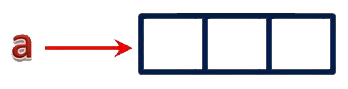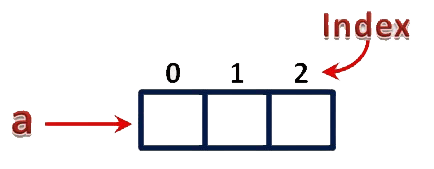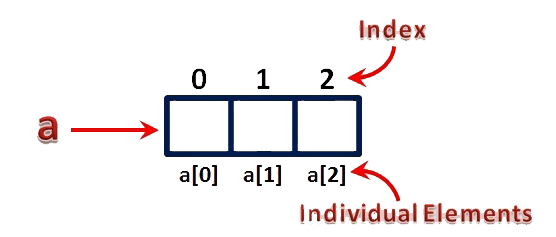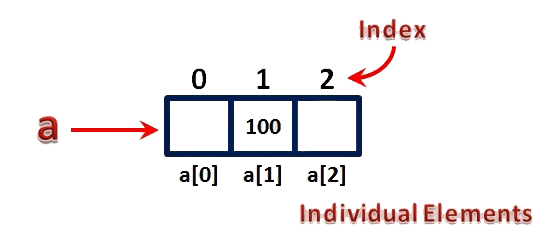The perfect place for easy learning...

×

# What is an Array?

Whenever we want to work with large number of data values, we need to use that much number of different variables. As the number of variables are increasing, complexity of the program also increases and programmers get confused with the variable names. There may be situations in which we need to work with large number of similar data values. To make this work more easy, C programming language provides a concept called "Array".

An array is a variable which can store multiple values of same data type at a time.

An array can also be defined as follows...

"Collection of similar data items stored in continuous memory locations with single name".

To understand the concept of arrays, consider the following example declaration.

#### int a, b, c;

Here, the compiler allocates 2 bytes of memory with name 'a', another 2 bytes of memory with name 'b' and more 2 bytes with name 'c'. These three memory locations are may be in sequence or may not be in sequence. Here these individual variables store only one value at a time.Now consider the following declaration...

#### int a;

Here, the compiler allocates total 6 bytes of continuous memory locations with single name 'a'. But allows to store three different integer values (each in 2 bytes of memory) at a time. And memory is organized as follows...That means all these three memory locations are named as 'a'. But "how can we refer individual elements?" is the big question. Answer for this question is, compiler not only allocates memory, but also assigns a numerical value to each individual element of an array. This numerical value is called as "Index". Index values for the above example are as follows...The individual elements of an array are identified using the combination of 'name' and 'index' as follows...

#### arrayName[indexValue]

For the above example, the individual elements can be referred as follows...If I want to assign a value to any of these memory locations (array elements), we can assign as follows...

#### a = 100;

The result will be as follows...There are different types of arrays in c programming language. Click on the below link to read TYPES OF ARRAYS